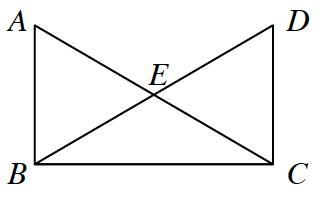### Home > GC > Chapter 8 > Lesson 8.1.5 > Problem8-58

8-58.

In the figure at right, $\overline{AB} \cong \overline{DC}$ and $\angle ABC \cong \angle DCB$.

1. Is $\overline{AC}\cong\overline{DB}$? Prove your answer using a flowchart or two-column proof.

Use one of the triangle congruence conjectures. Remember that $\overline{BC} \cong \overline{CB}$ because of the Reflexive property.

If you choose to prove your answer with a flowchart, remember to start with the three facts needed to use the appropriate congruence theorem.

2. Do the measures of $\angle ABC$ and $\angle DCB$ make any difference in your solution to part (a)? Explain why or why not.Explore using the eTool below.
Click the link to the right for full version. 8-63 HW eTool